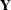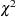# Overview: CANCORR Procedure

The CANCORR procedure performs canonical correlation, partial canonical correlation, and canonical redundancy analysis.

Canonical correlation is a generalization of multiple correlation for analyzing the relationship between two sets of variables. In multiple correlation, you examine the relationship between a linear combination of a set of explanatory variables,, and a single response variable,. In canonical correlation, you examine the relationship between linear combinations of the set ofvariables and linear combinations of a set ofvariables. These linear combinations are called canonical variables or canonical variates. Either set of variables can be considered explanatory or response variables, since the statistical model is symmetric in the two sets of variables. Simple and multiple correlation are special cases of canonical correlation in which one or both sets contain a single variable.

The CANCORR procedure tests a series of hypotheses that each canonical correlation and all smaller canonical correlations are zero in the population. PROC CANCORR uses an F approximation (Rao; 1973; Kshirsagar; 1972) that gives better small sample results than the usualapproximation. At least one of the two sets of variables should have an approximate multivariate normal distribution in order for the probability levels to be valid.

Both standardized and unstandardized canonical coefficients are computed, as well as the four canonical structure matrices showing correlations between the two sets of canonical variables and the two sets of original variables. A canonical redundancy analysis (Stewart and Love; 1968; Cooley and Lohnes; 1971) can also be done. PROC CANCORR provides multiple regression analysis options to aid in interpreting the canonical correlation analysis. You can examine the linear regression of each variable on the opposite set of variables.

PROC CANCORR can produce a data set containing the scores of each observation on each canonical variable, and you can use the PRINT procedure to list these values. A plot of each canonical variable against its counterpart in the other group is often useful, and you can use PROC SGPLOT with the output data set to produce these plots. A second output data set contains the canonical correlations, coefficients, and most other statistics computed by the procedure.